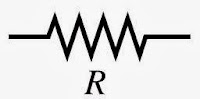### RESISTOR COLOR CODING

This is the first post of the section 'Hardware Basics', in this post we will look at few basic components we use frequently in our projects.

Resistor: Resistor is a two terminal component used almost in all the electronic devices, it resists the flow of current. Depending upon its value they resists(opposes) the flow of current.
Analogy: Speed breakers on road which resists the speed of vehicle, in the same way resistors resists the flow of current.
How it looks:
To know the value of the resister we should know how to read the colors which is printed on it.We will look how to 'color code' next.

Symbol:You can observe the symbol which is similar to "speed breaker of road" ;-)

COLOR CODING:
Every resistor printed with  different color bands and every color have its predefined value, to know the value of the resistor we should know color coding.

Step1: Find the silver or gold color on the resistor, it will be last color of the resistor(so check both the ends to find it), now hold its adjacent terminal with your left hand. It looks something like...

Step2: Looking from right, note the colors one after the other(numbered as 1,2,3 so on..)

Step3: Every color will have corresponding value(look at the below table). Calculate the value of register by

First color second color third color so on.. X 10^ last color(color before the silver color)

Example 1:

First color is Brown , next color red, next color black , next color black, next color silver or gold.

Values Brown=1, Red=2, Black=0, again Black=0

120x10^0 =120 (ohm)

Example 2:

Colors: Brown, black, orange

Brown=1, black=0, orange=3

10x10^3 = 10,000 = 10k ohm

Example 3:

Red=2, Red=2, Black=0
22x10^0 =220 ohm

Example 4:

violet=7 , green=5 , black=0
75x10^0= 750 ohm

Example 5:

100x10^0=1000=1k ohm

Tip to remember colors in order:
Just remember this phrase

BB ROY  Great Britain has Very Good Wife

B-Black=0
B-Brown=1
R-Red=2
O-Orange=3
Y-Yellow=4
G-Green=5
B-Blue=6
V-Violet=7
G-Gray=8
W-White=9

In our next post we will look at other components :-)

AUTHOR: YOUSTRON SIC

Sowpath das said...

This information here will surely be of some help to me : see more

shortcut keys of computer a to z said...

Get all the information of windows shortcut keys here on this site. And also get the instruction how to use windows shortcut keys.